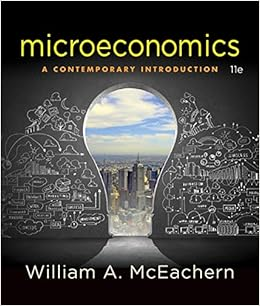# A shift in the aggregate demand curve starting from

• Notes
• 6
• 100% (7) 7 out of 7 people found this document helpful

This preview shows page 4 - 6 out of 6 pages.

##### We have textbook solutions for you!
The document you are viewing contains questions related to this textbook.The document you are viewing contains questions related to this textbook.
Chapter 6 / Exercise 8
Microeconomics: A Contemporary Introduction
McEachernExpert Verified
19.A shift in the aggregate demand curve, starting from long-run equilibrium, which increases output in the short run, will ______ in the long run, as compared to a short-run equilibrium.A)increase both output and the price levelB)decrease output but increase pricesC)increase output but decrease the price levelD)decrease both output and the price level
20.If the short-run IS-LMequilibrium occurs at a level of income below the natural level of output, then in the long run the price level will ______, shifting the ______ curve to the right and returning output to the natural level.
Page 4
##### We have textbook solutions for you!
The document you are viewing contains questions related to this textbook.The document you are viewing contains questions related to this textbook.
Chapter 6 / Exercise 8
Microeconomics: A Contemporary Introduction
McEachernExpert Verified
Use the following to answer questions 21-22:(Exhibit: Short Run to Long Run)21.(Exhibit: Short Run to Long Run) Based on the graph, if the economy starts from a short-term equilibrium at A, then the long-run equilibrium will be at ____ with a _____ price level.
22.(Exhibit: Short Run to Long Run) Based on the graph, if the economy starts from a short-term equilibrium at D, then the long-run equilibrium will be at ____ with a _____ price level.
23.The spending hypothesis suggests that the Great Depression was caused by a:A)leftward shift in the IScurve.B)rightward shift in the IScurve.C)leftward shift in the LMcurve.D)rightward shift in the LMcurve.
24.Allof the following events are consistent with the spending hypothesis as contributing to the Great Depression except:
Page 5
25.The money hypothesis suggests that the Great Depression was caused by a:
Page 6
•••